# AP Calculus BC Question 87: Answer and Explanation

### Test Information

Question: 87

5.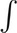sec4 x dx =

• A. tan4 x + C
• B. tan x +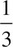tan3 x + C
• C. tan2 x + C
• D.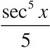+ C

Explanation:

B First, break up the integrand:sec4 x dx =(sec2 x)(sec2 x) dx.

Next, use the trig identity 1 + tan2 x = sec2 x to rewrite the integral.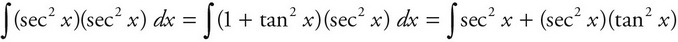We can evaluate these integrals separately.

The left one is easy:sec2 x dx = tan x + C.

We will use u-substitution for the right one. Let u = tan x and du = sec2 x dx. Then, substitute into the integral and integrate: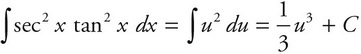.

Now, substitute back:tan3 x + C.

Combine the two integrals to get tan x +tan3 x + C.